常见排序算法及实现 原

2019独角兽企业重金招聘Python工程师标准>>>一、冒泡排序

冒泡排序（Bubble Sort）是先从数组第一个元素开始，依次比较相邻两个数，若前者比后者

这个算法的名字由来是因为越大的元素会经由交换慢慢“浮”到数列的顶端，故名。

1、算法原理

冒泡排序算法的运作如下：（从后往前）

1）比较相邻的元素。如果第一个比第二个大，就交换它们两个。

2）对每一对相邻元素作同样的工作，从开始第一对到结尾的最后一对。在这一点，最后的元

素应该会是最大的数。

3）针对所有的元素重复以上的步骤，除了最后一个。

4）持续每次对越来越少的元素重复上面的步骤，知道没有任何一对数字需要比较。

2、算法分析

时间复杂度：

若文件的初始状态是正序的，一趟扫描即可完成排序。所需的的关键字比较次数为最小n-1和

若初始文件是反序的，需要进行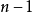趟排序。每趟排序要进行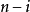次关键字的比较(1≤i≤n-

1)，且每次比较都必须移动记录三次来达到交换记录位置。在这种情况下，比较和移动次数均

因此，冒泡排序总的平均时间复杂度为O(n^2)。

算法稳定性：

（稳定性的解释：假定在待排序的记录序列中，存在多个具有相同的关键字的记录，若经过排

冒泡排序就是把小的元素往前调或者把大的元素往后调。比较是相邻的两个元素比较，交换

3、算法实现

``````/*
冒泡排序
*/

#include <iostream>
#include <stdio.h>

void bubble_sort( int *array, int length )
{
if( array == NULL || length <= 0 )
{
printf( "invalid input.\n" );
return;
}

int temp;

for( int i = 0; i < length - 1; i++ )
for( int j = 0; j < length - 1 - i; j++ )
{
if( array[ j ] > array[ j + 1 ] )
{
temp = array[ j ];
array[ j ] = array[ j + 1 ];
array[ j+ 1 ] = temp;
}
}
}

void Test( const char* testName, int *array, int length )
{
if( testName == NULL )
{
printf( "test invaild input.\n" );
return;
}

printf( "%s begins: \n", testName );

bubble_sort( array, length );

printf( "after bubble sort, the result is: \n" );
for( int i = 0; i < length; i++ )
printf( "%d ", array[ i ] );
printf( "\n" );
}

// 无重复数字
void Test1()
{
int length = 6;
int array[ ] = { 4, 2, 6, 7, 1, 5 };
Test( "Test1", array, length );
}

// 有重复数字
void Test2()
{
int length = 6;
int array[ ] = { 4, 2, 6, 2, 1, 5 };
Test( "Test2", array, length );
}

// 输入数组为空
void Test3()
{
Test( "Test3", NULL, -1 );
}

int main()
{
Test1();
Test2();
Test3();

return 0;
}``````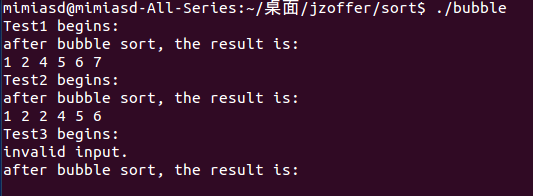二、选择排序

选择排序（Selection Sort）简单而低效。它线性逐一扫描数组元素，从中挑出最小的元素，

1、算法原理

n个记录的文件的直接选择排序可经过n-1趟直接选择排序得到有序结果：

1）初始状态：无序区为R[1...n]，有序区为空。

2）第一趟排序

在无序区R[1...n]中选出关键字最小的记录R[k]，将它与无序区的第一个记录R交换，使

R[1...1]和R[2...n]分别变为记录个数增加1个的新有序区和记录个数较少1个的新无序区。

......

3）第i趟排序

第i趟排序开始时，当前有序区和无序区分别为R[1...i-1]和R[i...n]。该趟排序从当前无序区中

2、算法分析

时间复杂度：

选择排序的交换操作介于 0 和 (n - 1） 次之间。选择排序的比较操作为 n (n - 1） / 2 次之间。

稳定性：

选择排序是给每个位置选择当前元素最小的，比如给第一个位置选择最小的，在剩余元素里面

9，我们知道第一遍选择第1个元素5会和2交换，那么原序列中两个5的相对前后顺序就被破坏

3、算法实现

``````/*
选择排序。
*/

#include <iostream>
#include <stdio.h>

void SelectSort( int *array, int length )
{
if( array == NULL || length <= 0 )
{
printf( "invaild input.\n" );
return;
}

int index = 0;

// 每次循环只进行一次交换，最多进行len - 1次循环，比冒泡进行交换的次数少。
for( int i = 0; i < length - 1; i++ )
{
// 第一次排序时，已经进行一次大循环，因此已经排好了1个元素
// 已排好序的元素0,...，i-2,i-1
// 待排元素为i，i+1,...，length-1

index = i;
for( int j = i + 1; j < length; j++ )
{
if( array[ j ] < array[ index ] )
{
index = j;
}
}

// 交换
if( index != i )
{
int temp = array[ i ];
array[ i ] = array[ index ];
array[ index ] = temp;
}
}
}

void Test( const char *testName, int *array, int length )
{
if( testName == NULL )
{
printf( "test invaild input.\n" );
return;
}

printf( "%s begins: \n", testName );

SelectSort( array, length );

printf( "after select sort, the result is: \n" );
for( int i = 0; i < length; i++ )
printf( "%d ", array[ i ] );
printf( "\n" );
}

// 无重复数字
void Test1()
{
int length = 6;
int array[ ] = { 4, 2, 6, 7, 1, 5 };
Test( "Test1", array, length );
}

// 有重复数字
void Test2()
{
int length = 6;
int array[ ] = { 4, 2, 6, 2, 1, 5 };
Test( "Test2", array, length );
}

// 输入数组为空
void Test3()
{
int array[ ] = {};
Test( "Test3", array, 0 );
}

// 输入数组为null，且长度异常
void Test4()
{
Test( "Test4", NULL, -1 );
}

int main()
{
Test1();
Test2();
Test3();
Test4();

return 0;
}``````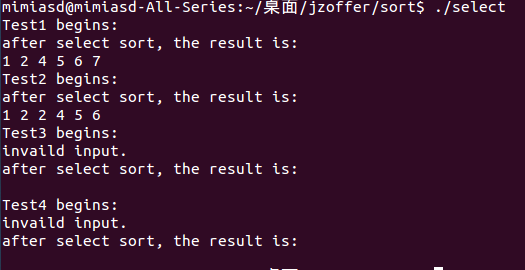三、归并排序

归并排序（Merge-Sort）是建立在归并操作上的一种有效的排序算法，该算法是采用分治法

（Divide and Conquer）的一个非常典型的应用。将数组分成两半，这两半分别排序后，再归

1、算法原理

归并操作的工作原理如下：

1）申请空间，使其大小为两个已经排序序列之和，该空间用来存放合并后的序列。

2）设定两个指针，最初位置分别为两个已经排序序列的起始位置。

3）比较两个指针所指向的元素，选择相对小的元素放入到合并空间，并移动指针到下一位

置。

4）重复步骤3直到某一指针超出序列尾，将另一序列剩下的所有元素直接复制到合并序列

尾。

2、算法分析

时间复杂度：

归并排序的效率是比较高的，设数列长为N，将数列分开成小数列一共要logN步，每一步都

稳定性：

归并排序是稳定的排序.即相等的元素的顺序不会改变.如输入记录 1(1) 3(2) 2(3) 2(4) 5(5) (括

3、算法实现

``````/*
归并排序。
*/

#include <iostream>
#include <stdio.h>

void merge( int *array, int low, int mid, int high )
{
int nLeft = low;  // nLeft是左边序列的下标
int nRight = mid + 1;  // nRight是右边序列的下标
int nMerge = 0; // nMerge是临时存放合并的下标

int *tempArray = ( int* )new int[ high - low + 1 ];  // tempArray是临时合并序列

// 扫描左边序列和右边序列，直到一边扫描结束
while( nLeft <= mid && nRight <= high )
{
if( array[ nLeft ] <= array[ nRight ] )
{
tempArray[ nMerge ] = array[ nLeft ];
nLeft++;
nMerge++;
}
else
{
tempArray[ nMerge ] = array[ nRight ];
nRight++;
nMerge++;
}
}

// 若左边序列还没扫描完，则将其全部赋值到临时合并序列
while( nLeft <= mid )
{
tempArray[ nMerge ] = array[ nLeft ];
nLeft++;
nMerge++;
}

// 若右边序列还没扫描完，则将其全部赋值到临时合并序列
while( nRight <= high )
{
tempArray[ nMerge ] = array[ nRight ];
nRight++;
nMerge++;
}

// 将临时合并序列复制到原始序列中
for( nMerge = 0, nLeft = low; nLeft <= high; nLeft++, nMerge++ )
{
array[ nLeft ] = tempArray[ nMerge ];
}

delete [] tempArray;
}

void mergeSort( int *array, int low, int high )
{
if( array == NULL || low < 0 || high < 0 || low > high )
{
printf( "invaild input.\n" );
return;
}

if( low < high )
{
int mid = ( low + high ) / 2 ;
mergeSort( array, low, mid );
mergeSort( array, mid + 1, high );
merge( array, low, mid, high );
}
}

void Test( const char* testName, int *array, int low, int high )
{
if( testName == NULL )
{
printf( "test invaild input.\n" );
return;
}

printf( "%s begins: \n", testName );

mergeSort( array, low, high );

printf( "after select sort, the result is: \n" );
for( int i = 0; i < high - low + 1; i++ )
printf( "%d ", array[ i ] );
printf( "\n" );
}

// 无重复数字
void Test1()
{
int length = 6;
int array[ ] = { 4, 2, 6, 7, 1, 5 };
Test( "Test1", array, 0, 5 );
}

// 有重复数字
void Test2()
{
int length = 6;
int array[ ] = { 4, 2, 6, 2, 1, 5 };
Test( "Test2", array, 0, 5 );
}

// 输入数字只有一个元素
void Test3()
{
int array[ ] = { 100 };
Test( "Test3", array, 0, 0 );
}

// 输入数组为空
void Test4()
{
int emptyArray[ ] = { };
Test( "Test4", emptyArray, 0, 0 );
}

// 输入数组为null，且长度异常
void Test5()
{
Test( "Test5", NULL, -1, -1 );
}

int main()
{
Test1();
Test2();
Test3();
Test4();
Test5();

return 0;
}``````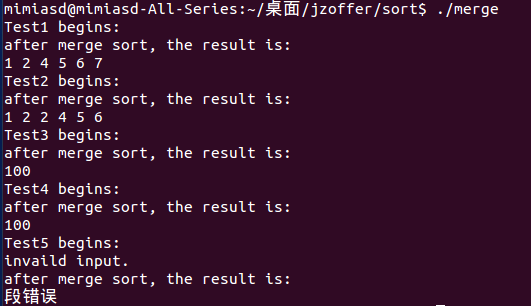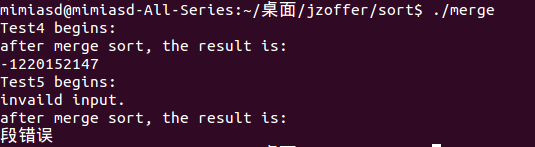（补充：1、当数组为空，low=0，high=0时，数组本应没有元素，但gcc编译后最终会打印出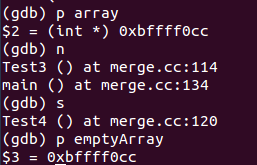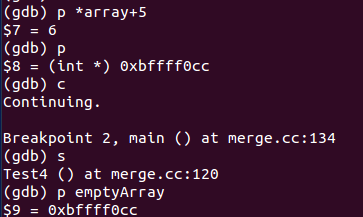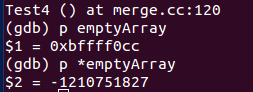（继续补充：好吧，没按捺住，又去找了为啥是上次Test结果的最后一个元素，可以从上图看

gcc数组内存分配策略有关，当然这里就不再跟下去了，要不然就没饭吃了╮(╯▽╰)╭ ）。

四、快速排序

快速排序（Quicksort）是对冒泡跑序的一种改进。它的基本思想是：随机选择一个元素，对

1、算法原理

基本思想：

1）先从数列中取出一个数作为基准数;

2）分区过程，将比这个数大的数全放到它的右边，小于或等于它的数全放到它的左边;

3）再对左右区间重复第二步，直到各区间只有一个数。

一趟快速排序的算法是：

1）设置两个变量i、j，排序开始的时侯：i=0，j=N-1;

2）以第一个数组元素作为关键数据，赋值给key，即key=A;

3）从j开始向前搜索，即由后开始向前搜索(j--)，找到第一个小于key的值A[j]，将A[j]和A[i]互

换;

4）从i开始向后搜索，即由前开始向后搜索(i++)，找到第一个大于key的A[i]，将A[i]和A[j]互换;

5）重复第3、4步，直到i=j。 （3,4步中，没找到符合条件的值，即3中A[j]不小于key,4中A[i]

不大于key的时候改变j、i的值，使得j=j-1，i=i+1，直至找到为止。找到符合条件的值，进

行交换的时候i， j指针位置不变。另外，i==j这一过程一定正好是i+或j-完成的时候，此时令

循环结束）。

快速排序还有很多改进版本，如随机选择基准数，区间内数据较少时直接用另外的方法排序以

2、算法分析

时间复杂度：

快速排序之所比较快，因为相比冒泡排序，每次交换是跳跃式的。每次排序的时候设置一个

稳定性：

快速排序有两个方向，左边的i下标一直往右走，当a[i] <= a[center_index]，其中center_index

a[center_index]。如果i和j都走不动了，i <= j, 交换a[i]和a[j],重复上面的过程，直到i>j。 交换a[j]

3、算法实现

第一种实现为选取第一个元素为枢纽：

``````/*
快速排序。（以第一个元素作为基准）
*/

#include <iostream>
#include <stdio.h>

void QuickSort( int *array, int low, int high )
{
if( array == NULL || low < 0 )
{
printf( "invalid input.\n" );
return;
}
if( high < 0 || low > high ) // 必须加这句，因为递归的时候high可能为-1
{
return;
}

int first = low;
int last = high;
int key = array[ first ]; // 用第一个元素作为作为枢纽

while( first < last )
{
while( first < last && array[ last ] >= key )
{
--last;
}

array[ first ] = array[ last ];  // 将比枢纽元素小的移到低端

while( first < last && array[ first ] <= key )
{
++first;
}

array[ last ] = array[ first ];  // 将比枢纽元素大的移到高端
}

array[ first ] = key; // 枢纽到位记录

QuickSort( array, low, first - 1 );
QuickSort( array, first + 1, high );

}

void Test( const char* testName, int* array, int low, int high )
{
if( testName == NULL )
{
printf( "test invaild input.\n" );
return;
}

printf( "%s begins: \n", testName );

QuickSort( array, low, high );

printf( "after quick sort, the result is: \n" );
for( int i = 0; i < high - low + 1; i++ )
printf( "%d ", array[ i ] );
printf( "\n" );
}

// 无重复数字
void Test1()
{
int array[ ] = { 4, 2, 6, 7, 1, 5 };
Test( "Test1", array, 0, 5 );
}

// 有重复数字
void Test2()
{
int array[ ] = { 4, 2, 6, 2, 1, 5 };
Test( "Test2", array, 0, 5 );
}

// 输入数字只有一个元素
void Test3()
{
int array[ ] = { 100 };
Test( "Test3", array, 0, 0 );
}

// 输入数组为空
void Test4()
{
int emptyArray[ ] = { };
Test( "Test4", emptyArray, 0, 0 );
}

// 输入数组为null，且长度异常
void Test5()
{
Test( "Test5", NULL, -1, -1 );
}

int main()
{
Test1();
Test2();
Test3();
Test4();
Test5();

return 0;
}``````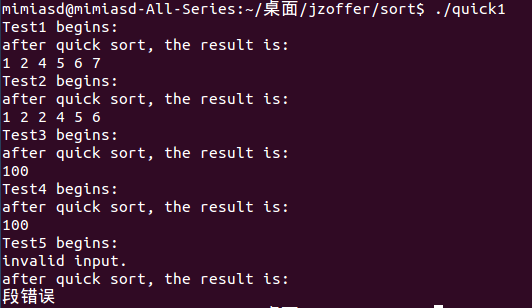``````/*
快速排序。（以随机元素作为基准）
*/

#include <stdlib.h>
#include <iostream>
#include <time.h>
#include <stdio.h>

void Swap( int *first, int *second )
{
int temp = *first;
*first = *second;
*second = temp;
}

int Partition( int *array, int low, int high )
{
if( array == NULL || low < 0 )
{
printf( "invalid input.\n" );
return -1;
}

srand( ( unsigned )time( NULL ) );     // 利用时间设置随机数种子
int index = low + rand()%( high - low + 1 ); // 产生low到high的随机数

Swap( &array[ index ], &array[ low ] );

int first = low;
int last = high;
int key = array[ first ]; // 用第一个元素作为作为枢纽

while( first < last )
{
while( first < last && array[ last ] >= key )
{
--last;
}

array[ first ] = array[ last ];  // 将比枢纽元素小的移到低端

while( first < last && array[ first ] <= key )
{
++first;
}

array[ last ] = array[ first ];  // 将比枢纽元素大的移到高端
}

array[ first ] = key; // 枢纽到位记录

return first;
}

/*
int Partition( int *array, int low, int high )
{
if( array == NULL || low < 0 )
{
printf( "invalid input.\n" );
return -1;
}

srand( ( unsigned )time( NULL ) );     // 利用时间设置随机数种子
int index = low + rand()%( high - low + 1 ); // 产生low到high的随机数

Swap( &array[ index ], &array[ low ] );

int small = low - 1;
for( index = low; index < high; ++index )
{
if( array[ index ] < array[ high ] )
{
++small;
if( small != index )
Swap( &array[ index ], &array[ small ] );
}
}

++small;
Swap( &array[ small ], &array[ high ] );

return small;
}
*/

void QuickSort( int *array, int low, int high )
{
if( low == high )
{
return;
}

int index = Partition( array, low, high );

if( index < 0 )
return;

if( index > low )
QuickSort( array, low, index - 1 );
if( index < high )
QuickSort( array, index + 1, high );
}

void Test( const char* testName, int* array, int low, int high )
{
if( testName == NULL )
{
printf( "test invaild input.\n" );
return;
}

printf( "%s begins: \n", testName );

QuickSort( array, low, high );

printf( "after quick sort, the result is: \n" );
for( int i = 0; i < high - low + 1; i++ )
printf( "%d ", array[ i ] );
printf( "\n" );
}

// 无重复数字
void Test1()
{
int array[ ] = { 4, 2, 6, 7, 1, 5 };
Test( "Test1", array, 0, 5 );
}

// 有重复数字
void Test2()
{
int array[ ] = { 4, 2, 6, 2, 1, 5 };
Test( "Test2", array, 0, 5 );
}

// 输入数字只有一个元素
void Test3()
{
int array[ ] = { 100 };
Test( "Test3", array, 0, 0 );
}

// 输入数组为空
void Test4()
{
int emptyArray[ ] = { };
Test( "Test4", emptyArray, 0, 0 );
}

// 输入数组为null，且长度异常
void Test5()
{
Test( "Test5", NULL, -1, -1 );
}

int main()
{
Test1();
Test2();
Test3();
Test4();
Test5();

return 0;
}``````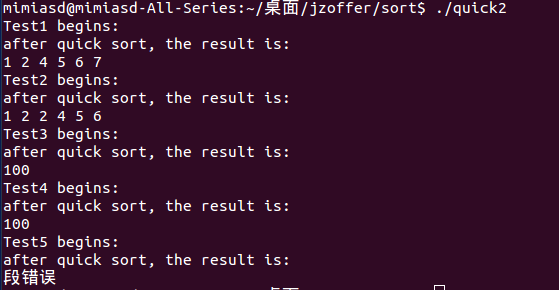随机枢纽快速排序的Partition函数有两种实现。

五、堆排序

堆排序(Heapsort)是指利用堆积树（堆）这种数据结构所设计的一种排序算法，它是选择排序

。大根堆的要求是每个节点的值都不大于其父节点的值，即A[PARENT[i]] >= A[i]。在数组的

堆实际上是一棵完全二叉树，其任何一非叶节点满足性质： Key[i]<=key[2i+1]&&Key[i]

<=key[2i+2]或者Key[i]>=Key[2i+1]&&key>=key[2i+2]即任何一非叶节点的关键字不大于或者不

key>=key[2i+2]称为大顶堆，满足 Key[i]<=key[2i+1]&&Key[i]<=key[2i+2]称为小顶堆。由上述性

1、算法原理

其基本思想（大顶堆）：

1）将初始待排序关键字序列(R1,R2....Rn)构建成大顶堆，此堆为初始的无序区；

2）将堆顶元素R与最后一个元素R[n]交换，此时得到新的无序区(R1,R2,......Rn-1)和新的有

3）由于交换后新的堆顶R可能违反堆的性质，因此需要对当前无序区(R1,R2,......Rn-1)调整

操作过程如下：

1）初始化堆：将R[1..n]构造为堆；

2）将当前无序区的堆顶元素R同该区间的最后一个记录交换，然后将新的无序区调整为新

2、算法分析

时间复杂度：

堆排序其实也是一种选择排序，是一种树形选择排序。只不过直接选择排序中，为了从

R[1...n]中选择最大记录，需比较n-1次，然后 从R[1...n-2]中选择最大记录需比较n-2次。事实上

稳定性:

我们知道堆的结构是节点i的孩子为2*i和2*i+1节点，大顶堆要求父节点大于等于其2个子节

3、算法实现

``````/*
堆排序。
*/

#include <iostream>
#include <stdio.h>

// array是待调整的堆数组，pos是待调整的数组元素的位置，length是数组的长度
// 本函数功能是：根据数组array构建大根堆
void HeapAdjust( int *array, int pos, int length )
{
if( array == NULL || pos < 0 || length <= 0 )
{
printf( "invalid input.\n" );
return;
}

int child;
int temp;

for( ; 2 * pos + 1 < length; pos = child )
{
child = 2 * pos + 1;  // 子结点的位置

if( child < length - 1 && array[ child + 1 ] > array[ child ] )  // 得到子结点中较大的结点
++child;

if( array[ pos ] < array[ child ] )  // 如果较大的子结点大于父结点那么把较大的子结点往上移动，替换它的父结点
{
temp = array[ pos ];
array[ pos ] = array[ child ];
array[ child ] = temp;
}
else
break;
}
}

void HeapSort( int *array, int length )
{
int i;

// 调整序列的前半部分元素，调整完之后第一个元素是序列的最大的元素
// length/2-1是最后一个非叶节点
for( i = length / 2 - 1; i >= 0; --i )

// 从最后一个元素开始对序列进行调整，不断的缩小调整的范围直到第一个元素
for( i = length - 1; i > 0; --i )
{
// 把第一个元素和当前的最后一个元素交换，
// 保证当前的最后一个位置的元素都是在现在的这个序列之中最大的
array[ i ] = array[ 0 ] ^ array[ i ];
array[ 0 ] = array[ 0 ] ^ array[ i ];
array[ i ] = array[ 0 ] ^ array[ i ];

// 不断缩小调整heap的范围，每一次调整完毕保证第一个元素是当前序列的最大值
}
}

void Test( const char* testName, int* array, int length )
{
if( testName == NULL )
{
printf( "test invaild input.\n" );
return;
}

printf( "%s begins: \n", testName );

HeapSort( array, length );

printf( "after heap sort, the result is: \n" );
for( int i = 0; i < length; i++ )
printf( "%d ", array[ i ] );
printf( "\n" );
}

// 无重复数字
void Test1()
{
int array[ ] = { 4, 2, 6, 7, 1, 5 };
Test( "Test1", array, 6 );
}

// 有重复数字
void Test2()
{
int array[ ] = { 4, 2, 6, 2, 1, 5 };
Test( "Test2", array, 6 );
}

// 输入数字只有一个元素
void Test3()
{
int array[ ] = { 100 };
Test( "Test3", array, 1 );
}

// 输入数组为空
void Test4()
{
int emptyArray[ ] = { };
Test( "Test4", emptyArray, 0 );
}

// 输入数组为null，且长度异常
void Test5()
{
Test( "Test5", NULL, -1 );
}

int main()
{
Test1();
Test2();
Test3();
Test4();
Test5();

return 0;
}``````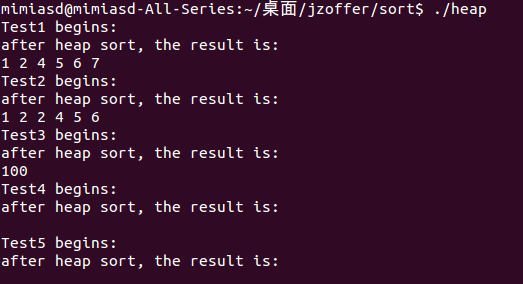六、基数排序

1、算法原理

1）首先根据个位数的数值，在走访数值时将它们分配至编号0到9的桶子中，接下来将这些桶

2）接着再进行一次分配，这次是根据十位数来分配，接下来将这些桶子中的数值重新串接起

这时候整个数列已经排序完毕；如果排序的对象有三位数以上，则持续进行以上的动作直至

最高位优先(Most Significant Digit first)法，简称MSD法：先按k1排序分组，同一组中记录，

最低位优先(Least Significant Digit first)法，简称LSD法：先从kd开始排序，再对kd-1进行排

2、算法分析

稳定性:

基数排序是按照低位先排序，然后收集；再按照高位排序，然后再收集；依次类推，直到最高

3、算法实现

``````/*
基数排序。
*/

#include <iostream>
#include <stdio.h>

// 辅助函数，求数据的最大位数
int MaxBit( int *array, int n )
{
int maxbit = 1;  // 保存最大的位数
int div = 10;

for( int i = 0; i < n; ++i )
{
while( array[ i ] >= div )
{
div *= 10;
++maxbit;
}
}

return maxbit;
}

void RadixSort( int *array, int n )
{
if( array == NULL || n < 0 )
{
printf( "invalid input.\n" );
return;
}

int maxbit = MaxBit( array, n );
int *temp = new int[ n ];
int *count = new int[ 10 ];  // 计数器
int i, j, k;

for( i = 1; i <= maxbit; i++ )  //进行maxbit次排序
{
for( j = 0; j < 10; j++ )
count[ j ] = 0;     // 每次分配前清空计数器

for( j = 0; j < n; j++ )
{
k = ( array[ j ] / radix ) % 10;   // 统计每个桶中的记录数
count[ k ]++;
}

for( j = 1; j < 10; j++ )
count[ j ] = count[ j - 1 ] + count[ j ];  // 将tmp中的位置依次分配给每个桶

for( j = n - 1; j >= 0; j-- )    // 将所有桶中记录依次收集到tmp中
{
k = ( array[ j ] / radix ) % 10;
temp[ count[ k ] - 1 ] = array[ j ];
count[ k ]--;
}

for( j = 0; j < n; j++ )  // 将临时数组的内容复制到data中
array[ j ] = temp[ j ];

}

delete [] temp;
delete [] count;
}

void Test( const char* testName, int* array, int n )
{
if( testName == NULL )
{
printf( "test invaild input.\n" );
return;
}

printf( "%s begins: \n", testName );

printf( "after radix sort, the result is: \n" );
for( int i = 0; i < n; i++ )
printf( "%d ", array[ i ] );
printf( "\n" );
}

// 无重复数字
void Test1()
{
int array[ ] = { 42, 24, 61, 71, 11, 57 };
Test( "Test1", array, 6 );
}

// 有重复数字
void Test2()
{
int array[ ] = { 421, 24, 6,421, 121, 54 };
Test( "Test2", array, 6 );
}

// 输入数字只有一个元素
void Test3()
{
int array[ ] = { 100 };
Test( "Test3", array, 1 );
}

// 输入数组为空
void Test4()
{
int emptyArray[ ] = { };
Test( "Test4", emptyArray, 0 );
}

// 输入数组为null，且长度异常
void Test5()
{
Test( "Test5", NULL, -1 );
}

int main()
{
Test1();
Test2();
Test3();
Test4();
Test5();

return 0;
}``````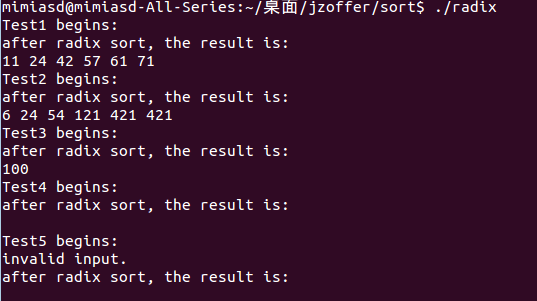thanatos_y

PHP常见排序算法学习

moTzxx
2017/10/27
0
0
[仅供个人参考系列]个人整理基础的排序、查找等算法相关笔记

1.常见排序算法的时间、空间复杂度 2.具体算法的实现 快速排序（根据定位pivot元素的方法，分为简单版和技巧版） 简单版： 复杂版：（请参考剑指offer上79页的相关写法） 直接插入算法： 堆排...

jayxujia123
2018/03/28
0
0
PHP常见排序算法整理学习

u011415782
2017/10/24
0
0
JavaScript实现经典排序算法

Luther_Li
04/08
0
0

2018/09/03
0
0

# -*- coding: utf-8 -*-"""Created on Thu Apr 18 17:05:47 2019@author: HeyJude"""import timestart_time = time.time()def GetText(pic_path, text_path): import pytesser......

KYO4321
12分钟前
1
0
Java多线程之创建线程的三种方式比较

16分钟前
0
0
inner join 与 left join 之间的区别

dragon_tech
16分钟前
1
0
linux下cpio.gz文件的解压方法

linux下cpio.gz文件的解压方法 linux下cpio.gz文件的解压方法linux解压cpiocpio.gz 今天下载了 10201_database_linux_x86_64.cpio.gz 文件，解压方法如下： 1. gunzip 10201_database_linux...

19分钟前
1
0
Shell分析服务器日志，解锁各种新姿势

Shell分析服务器日志，解锁各种新姿势 DevOps技术栈 5月10日 作者：Panda 原文：https://segmentfault.com/a/1190000009745139 自己的小网站跑在阿里云的ECS上面,偶尔也去分析分析自己网站服...

linzhuangrong
21分钟前
2
0